MathScore EduFighter is one of the best math games on the Internet today. You can start playing for free!

## Probability 2 - Sample Math Practice Problems

The math problems below can be generated by MathScore.com, a math practice program for schools and individual families. References to complexity and mode refer to the overall difficulty of the problems as they appear in the main program. In the main program, all problems are automatically graded and the difficulty adapts dynamically based on performance. Answers to these sample questions appear at the bottom of the page. This page does not grade your responses.

See some of our other supported math practice problems.

### Complexity=5

Find the probability that if you randomly select one piece from each of the three shapes, you will get 3 highlighted pieces and the odds of getting at least one unhighlighted piece.

1.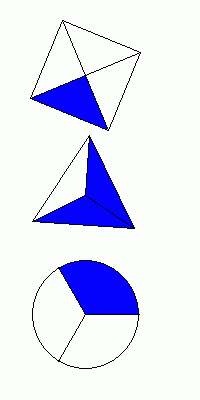Probability: Odds:

2.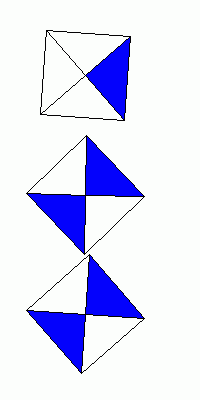Probability: Odds:

### Complexity=6

Find the probability that if you randomly select one piece from each of the three shapes, you will get 3 highlighted pieces and the odds of getting at least one unhighlighted piece.

1.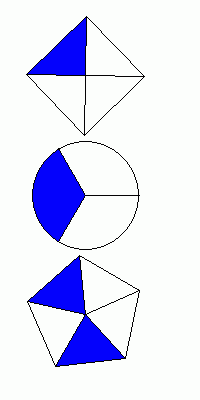Probability: Odds:

2.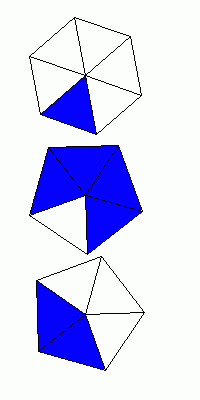Probability: Odds:

### Complexity=7

Find the probability that if you randomly select one piece from each of the three shapes, you will get 3 highlighted pieces and the odds of getting at least one unhighlighted piece.

1.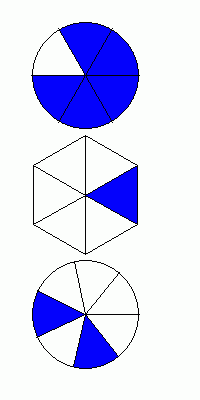Probability: Odds:

2.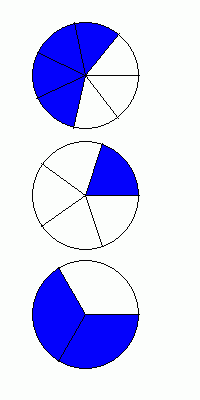Probability: Odds:

### Complexity=8

Find the probability that if you randomly select one piece from each of the three shapes, you will get 3 highlighted pieces and the odds of getting at least one unhighlighted piece.

1.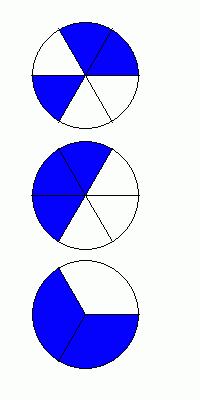Probability: Odds:

2.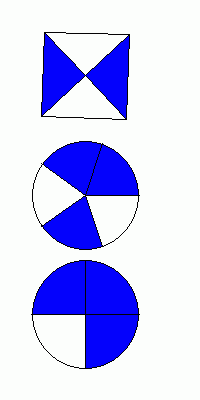Probability: Odds:

### Complexity=9

Find the probability that if you randomly select one piece from each of the three shapes, you will get 3 highlighted pieces and the odds of getting at least one unhighlighted piece.

1.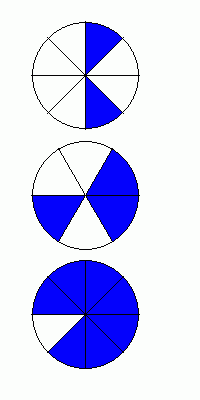Probability: Odds:

2.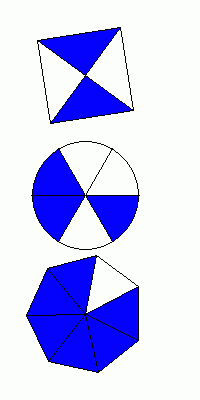Probability: Odds:

### Complexity=10

Find the probability that if you randomly select one piece from each of the three shapes, you will get 3 highlighted pieces and the odds of getting at least one unhighlighted piece.

1.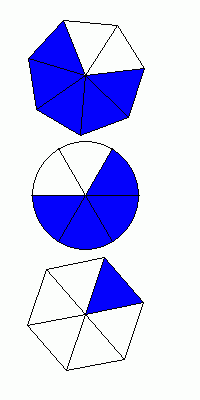Probability: Odds:

2.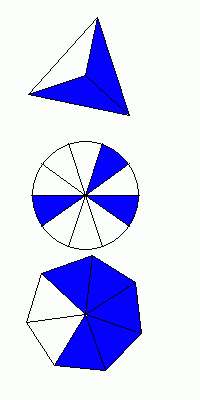Probability: Odds:

### Complexity=5

Find the probability that if you randomly select one piece from each of the three shapes, you will get 3 highlighted pieces and the odds of getting at least one unhighlighted piece.

1Probability: Odds:

Solution
Probability that all 3 pieces are highlighted:
P(1st piece highlighted) = 1/4.
P(2nd piece highlighted) = 2/3.
P(3rd piece highlighted) = 1/3.
P(all highlighted) = 1/4 × 2/3 × 1/3 = 1/18.

Odds of choosing at least one unhighlighted piece:
P(at least one piece unhighlighted) = 1 - P(all highlighted) = 1 - 1/18 = 17/18.
Odds(at least one piece unhighlighted) = 17:(18 - 17) = 17:1.
2Probability: Odds:

Solution
Probability that all 3 pieces are highlighted:
P(1st piece highlighted) = 1/4.
P(2nd piece highlighted) = 2/4 = 1/2.
P(3rd piece highlighted) = 2/4 = 1/2.
P(all highlighted) = 1/4 × 1/2 × 1/2 = 1/16.

Odds of choosing at least one unhighlighted piece:
P(at least one piece unhighlighted) = 1 - P(all highlighted) = 1 - 1/16 = 15/16.
Odds(at least one piece unhighlighted) = 15:(16 - 15) = 15:1.

### Complexity=6

Find the probability that if you randomly select one piece from each of the three shapes, you will get 3 highlighted pieces and the odds of getting at least one unhighlighted piece.

1Probability: Odds:

Solution
Probability that all 3 pieces are highlighted:
P(1st piece highlighted) = 1/4.
P(2nd piece highlighted) = 1/3.
P(3rd piece highlighted) = 2/5.
P(all highlighted) = 1/4 × 1/3 × 2/5 = 1/30.

Odds of choosing at least one unhighlighted piece:
P(at least one piece unhighlighted) = 1 - P(all highlighted) = 1 - 1/30 = 29/30.
Odds(at least one piece unhighlighted) = 29:(30 - 29) = 29:1.
2Probability: Odds:

Solution
Probability that all 3 pieces are highlighted:
P(1st piece highlighted) = 1/6.
P(2nd piece highlighted) = 4/5.
P(3rd piece highlighted) = 2/5.
P(all highlighted) = 1/6 × 4/5 × 2/5 = 4/75.

Odds of choosing at least one unhighlighted piece:
P(at least one piece unhighlighted) = 1 - P(all highlighted) = 1 - 4/75 = 71/75.
Odds(at least one piece unhighlighted) = 71:(75 - 71) = 71:4.

### Complexity=7

Find the probability that if you randomly select one piece from each of the three shapes, you will get 3 highlighted pieces and the odds of getting at least one unhighlighted piece.

1Probability: Odds:

Solution
Probability that all 3 pieces are highlighted:
P(1st piece highlighted) = 5/6.
P(2nd piece highlighted) = 1/6.
P(3rd piece highlighted) = 2/7.
P(all highlighted) = 5/6 × 1/6 × 2/7 = 5/126.

Odds of choosing at least one unhighlighted piece:
P(at least one piece unhighlighted) = 1 - P(all highlighted) = 1 - 5/126 = 121/126.
Odds(at least one piece unhighlighted) = 121:(126 - 121) = 121:5.
2Probability: Odds:

Solution
Probability that all 3 pieces are highlighted:
P(1st piece highlighted) = 4/7.
P(2nd piece highlighted) = 1/5.
P(3rd piece highlighted) = 2/3.
P(all highlighted) = 4/7 × 1/5 × 2/3 = 8/105.

Odds of choosing at least one unhighlighted piece:
P(at least one piece unhighlighted) = 1 - P(all highlighted) = 1 - 8/105 = 97/105.
Odds(at least one piece unhighlighted) = 97:(105 - 97) = 97:8.

### Complexity=8

Find the probability that if you randomly select one piece from each of the three shapes, you will get 3 highlighted pieces and the odds of getting at least one unhighlighted piece.

1Probability: Odds:

Solution
Probability that all 3 pieces are highlighted:
P(1st piece highlighted) = 3/6 = 1/2.
P(2nd piece highlighted) = 3/6 = 1/2.
P(3rd piece highlighted) = 2/3.
P(all highlighted) = 1/2 × 1/2 × 2/3 = 1/6.

Odds of choosing at least one unhighlighted piece:
P(at least one piece unhighlighted) = 1 - P(all highlighted) = 1 - 1/6 = 5/6.
Odds(at least one piece unhighlighted) = 5:(6 - 5) = 5:1.
2Probability: Odds:

Solution
Probability that all 3 pieces are highlighted:
P(1st piece highlighted) = 2/4 = 1/2.
P(2nd piece highlighted) = 3/5.
P(3rd piece highlighted) = 3/4.
P(all highlighted) = 1/2 × 3/5 × 3/4 = 9/40.

Odds of choosing at least one unhighlighted piece:
P(at least one piece unhighlighted) = 1 - P(all highlighted) = 1 - 9/40 = 31/40.
Odds(at least one piece unhighlighted) = 31:(40 - 31) = 31:9.

### Complexity=9

Find the probability that if you randomly select one piece from each of the three shapes, you will get 3 highlighted pieces and the odds of getting at least one unhighlighted piece.

1Probability: Odds:

Solution
Probability that all 3 pieces are highlighted:
P(1st piece highlighted) = 2/8 = 1/4.
P(2nd piece highlighted) = 3/6 = 1/2.
P(3rd piece highlighted) = 7/8.
P(all highlighted) = 1/4 × 1/2 × 7/8 = 7/64.

Odds of choosing at least one unhighlighted piece:
P(at least one piece unhighlighted) = 1 - P(all highlighted) = 1 - 7/64 = 57/64.
Odds(at least one piece unhighlighted) = 57:(64 - 57) = 57:7.
2Probability: Odds:

Solution
Probability that all 3 pieces are highlighted:
P(1st piece highlighted) = 2/4 = 1/2.
P(2nd piece highlighted) = 3/6 = 1/2.
P(3rd piece highlighted) = 6/7.
P(all highlighted) = 1/2 × 1/2 × 6/7 = 3/14.

Odds of choosing at least one unhighlighted piece:
P(at least one piece unhighlighted) = 1 - P(all highlighted) = 1 - 3/14 = 11/14.
Odds(at least one piece unhighlighted) = 11:(14 - 11) = 11:3.

### Complexity=10

Find the probability that if you randomly select one piece from each of the three shapes, you will get 3 highlighted pieces and the odds of getting at least one unhighlighted piece.

1Probability: Odds:

Solution
Probability that all 3 pieces are highlighted:
P(1st piece highlighted) = 5/7.
P(2nd piece highlighted) = 4/6 = 2/3.
P(3rd piece highlighted) = 1/6.
P(all highlighted) = 5/7 × 2/3 × 1/6 = 5/63.

Odds of choosing at least one unhighlighted piece:
P(at least one piece unhighlighted) = 1 - P(all highlighted) = 1 - 5/63 = 58/63.
Odds(at least one piece unhighlighted) = 58:(63 - 58) = 58:5.
2Probability: Odds:

Solution
Probability that all 3 pieces are highlighted:
P(1st piece highlighted) = 2/3.
P(2nd piece highlighted) = 3/10.
P(3rd piece highlighted) = 5/7.
P(all highlighted) = 2/3 × 3/10 × 5/7 = 1/7.

Odds of choosing at least one unhighlighted piece:
P(at least one piece unhighlighted) = 1 - P(all highlighted) = 1 - 1/7 = 6/7.
Odds(at least one piece unhighlighted) = 6:(7 - 6) = 6:1.